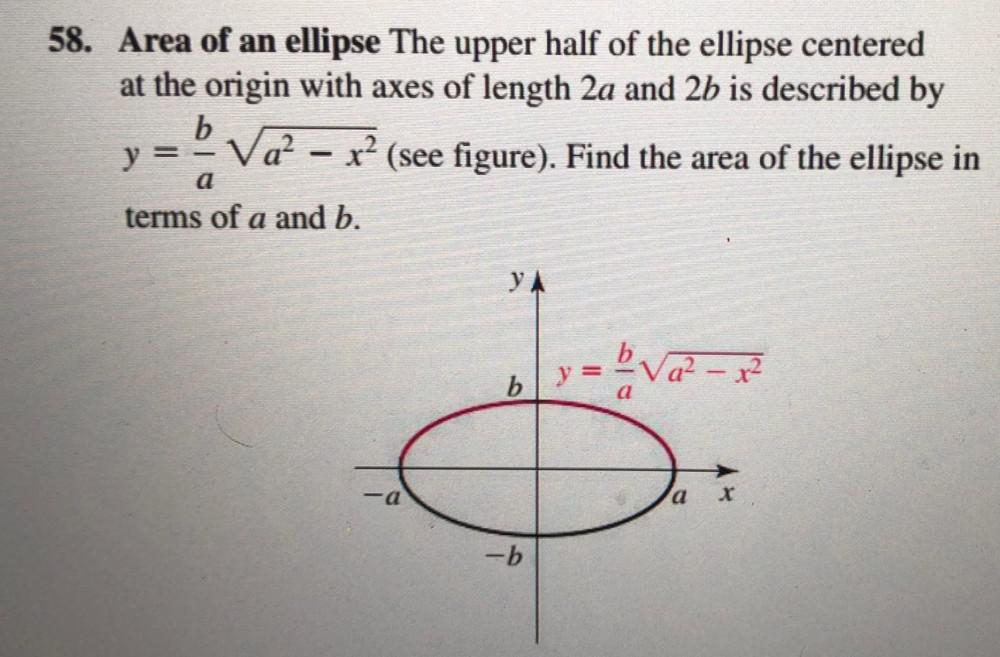Question:

# 58. Area of an ellipse The upper half of the ellipse centered at the origin with axes of length 2a and 2b is described by b y =58. Area of an ellipse The upper half of the ellipse centered at the origin with axes of length 2a and 2b is described by b y = V22 - x? (see figure). Find the area of the ellipse in Va² ( terms of a and b. - a YA y = ba2-x² b = a ) -a X -b